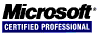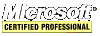# Intel IA-32 Assembly Tutorial

## Conditional Processing

#### Boolean and Comparison Instructions

Boolean instructions `AND`, `OR`, `XOR`, `NOT`, `TEST`, `BT`, `BTC`, `BTR`, and `BTS`. Each of these instructions affects the CPU flags:

• The Zero Flag is set when the result of an operation equals zero.
• The Carry Flag is set when an instruction generates a result that is too large or too small for the destination operand.
• The Sign Flag is a copy of the high bit of the destination operand, indicating that it is negative is set or positive if clear.
• The Overflow Flag is set when an instruction generates and invalid signed result.
• The Parity Flag is set when an instruction generates an even number of 1 bits in the low byte of the destination operand.

The `AND` instruction performs a boolean (bitwise) AND operation between each pair of matching bits in two operands and places the result in the destination operand:

``````	;usage: AND destination,source
mov eax,01101010b
and eax,00001000b ;EAX = 8
mov eax,01101010b
and eax,00000100b ;EAX = 0

;    10111000
;AND 10101001
;    --------
;    10101000
``````

The `AND` instruction always clears the Overflow and Carry Flags. It modifies the Sign, Zero and Parity Flags according to the value of the destination operand.

The `OR` instruction performs a boolean OR operation between each pair of matching bits in two operands and places the result in the destination operand:

``````	;usage: OR destination,source
mov eax,01101010b
or  eax,00001001b ;EAX = 01101011b (6Bh)
mov eax,00001000b
or  eax,00000100b ;EAX = 12

;   10111000
;OR 10101001
;   --------
;   10111001
``````

The `OR` instruction always clears the Carry and Overflow Flags. It modifies the Sign, Zero and Parity Flags according to the value of the destination operand. For example, you can `OR` a number with itself or zero to obtain information about its value. For example:

``````	or ebx,ebx
``````
 Zero Flag Sign Flag Then value in EBX... clear clear is greater than zero set clear is equal to zero clear set is less than zero

The `XOR` instruction performs a boolean exclusive-OR operation between each pair of matching bits in two operands, and stores the result in the destination operand:

``````
;usage: XOR destination,source
mov eax,01101010b
xor eax,00001001b ;EAX = 01100011b (63h)
mov eax,10001000b
xor eax,10000100b ;EAX = 12

;    10111000 <-- original bits
;XOR 10101001
;    --------
;    00010001
;XOR 10101001 <-- XOR the same value again
;    --------
;    10111000 <-- returns to original bits
``````

The `XOR` instruction always clears the Overflow and Carry Flags. It modifies the Sign, Zero and Parity Flags according to the value of the destination operand.

The Parity Flag indicates whether the lowest byte of the result of a bitwise or arithmetic operation has an even or odd number of 1 bits. The flag is set when the parity is even. It is clear when the parity is odd. One way to check the parity of a number without changing its value is to `XOR` the number with zero:

``````	mov al,10010111b    ;5 bits is odd parity
xor al,0            ;Parity Flag clear
mov al,10011001b    ;4 bits is even parity
xor al,0            ;Parity Flag set
``````

The `NOT` instruction toggles all bits in an operand:

``````	mov eax,00001111b
not eax             ;EAX = F0h (11110000b)
``````

No flags are affected by the `NOT` instruction.

The `TEST` instruction performs an implied AND operation with the only difference being that `TEST` does not modify the destination operation. It only sets the flags accordingly.

```	01010101 <-- input value
00001101 <-- TEST value
00000101 <-- Zero Flag (ZF)=0
01010101 <-- input value
10000010 <-- TEST value
00000000 <-- Zero Flag (ZF) = 1
```

The `TEST` instruction always clears the Overflow and Carry flags. It modifies the Sign, Zero and Parity Flags just like the `AND` instruction.

The `CMP` (compare) instruction performs an implied subtraction of a source operand from a destination operand and neither operand is modified.

``````	;CMP destination,source
cmp eax,2           ;EAX is not modified
``````

`CMP` uses the same operand combinations as the `AND` instruction.

The `CMP` instruction changes the Overflow, Sign, Zero, Carry, Auxiliary Carry and Parity Flags according to the value the destination operand would have had if the `SUB` instruction were used.

 CMP Results Zero Flag Carry Flag destination < source 0 1 destination > source 0 0 destination = source 1 0

If the two operands being compared are assumed to be signed then the Sign, Zero and Overflow Flags indicate the following relations between operands.

 CMP Results Flags destination < source SF ≠ OF destination > source SF = OF destination = source ZF = 1

`CMP` provides the basis for most conditional logic structures. When a conditional jump instruction follows `CMP`, the result is a higher-level language IF statement.

<< < [Page 9 of 14] > >>